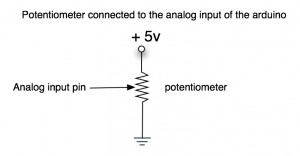I got myself a rotary potentiometer at dx.com and decided to come up with a basic article.

First, lets have a quick look at the below schematic to understand how a potentiometer works.Now, lets plug it to our arduino and while we are it, lets use its retrieved value (thru analogread) to dim a led (thru analogwrite).Now lets have a look at the arduino sketch

```byte potPin=0; //Analog 0 connected to the potentiometer
byte LEDPin=6; //Connected to LED on Pin 6
float potValue=0; //Value returned from the potentiometer
float v=0; //voltage (0-5v)

void setup(){
Serial.begin(9600);
pinMode(LEDPin, OUTPUT); //Set Pin 6 as an Output
}

void loop(){

potValue = analogRead(potPin)/4; //Read the potentiometer, convert it to 0 - 255
Serial.println(potValue,0);
v=potValue*5/255; //to calculate the voltage send out on pin6
Serial.println(v, 2);
analogWrite(LEDPin, potValue); //Write the converted potentiometer value to LED pin
delay(100);
}
```

here we go : rotate your potentiometer and see your led dim in and out

Ce site utilise Akismet pour réduire les indésirables. En savoir plus sur comment les données de vos commentaires sont utilisées.